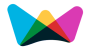##Online,Easy and Quick Student Education Loan EMI Repayment Calculation#Student Education Loan Calculator

Instantly calculates your education loan EMI and total loan repayment for different periods.

Months
Repayment Term Calculation
1 to 5 Years   6 to 10 Years   11 to 15 Years### Student Education Loan Calculation, formulas

Student Education Loan calculator is an EMI repayment calculator which helps you calculate your monthly repayments.

(All you have to do is, Just enter the educational loan amount, Period (in months) and the annual rate of interest, and you are done.)

The Education repayment loan calculator uses the following formulas:

EMI = ( P × r × (1+r)n ) / ((1+r)n - 1)

Total Loan Repayment = EMI × n

Where,

EMI = Equated Monthly Installment

r = Interest rate / 1200

P = Loan Amount

n = Period

###More Calculators ►

Weekly Interest Calculator

Short Term Interest Calculator

Savings Interest Calculator